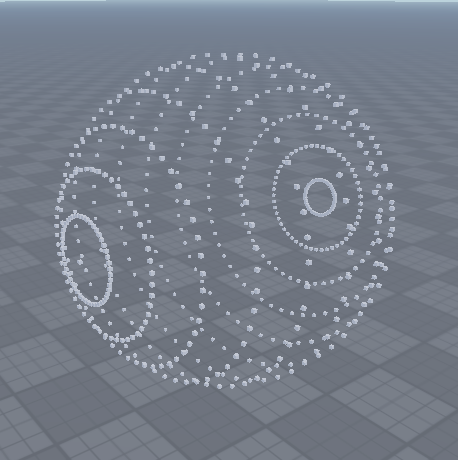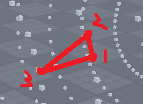# Triangulation Help

So im currently trying to make a deformable ball and I have everything working except how to define where to draw the triangles, all the triangulation algorithms I have found are 2d, could anyone lend some help?

3 Likes

For a sphere you can just use any equation that can give you points on the sphere then draw triangles between those points.

The parametric equation of a sphere is:
`x = r * cos(a) * sin(b)`
`y = r * sin(a) * sin(b)`
`z = r * cos(b)`

where `a` is the longitude and `b` is the azimuthal angle. (iirc this is just the colatitude angle)

Here is some example code:
`r` is the radius
`n` is the amount of point per “band” of the sphere.

``````local r = 5
local n = 25

local function map(i, a1, a2, b1, b2)
return b1 + (i - a1) * (b2 - b1) / (a2 - a1)
end

local function point(x, y, z)
local part = Instance.new("Part")

part.Anchored = true
part.Size = Vector3.new(0.1, 0.1, 0.1)
part.CFrame = CFrame.new(x, y, z)
part.Parent = workspace
end

for i = 1, n do
local a = map(i, 0, n, -math.pi, math.pi)

for j = 1, n do
local b = map(j, 0, n, -math.pi / 2, math.pi / 2)

local x = r * math.sin(a) * math.cos(b)
local y = r * math.sin(a) * math.sin(b)
local z = r * math.cos(a)

point(x, r + 5 + y, z)
end
end
``````

You will notice that the points are not all equidistant so the sphere looks “weird”.Here is a pdf about placing points on a sphere so that they are as close as you can get to them being equidistant.

5 Likes

I think that you have 2 options:

1. Maybe you can transform the 3D representation of your points to a 2D one and then apply the triangulation.
2. Write a triangulation module that can work with 3D data.

Drawing a sphere made of triangles is something that I have done before. I used the free 2D Delaunay Triangulation module from the ToolBox and ported my 3D points to a 2D representation (you can imagine it as cutting a sphere by 1/4th and folding it to a plane). Then I fed that data to the triangulation algorithm and it worked.

I already have a function to generate points on a sphere its the problem of figuring out where to draw triangles. Hence I need a 3d triangulation algorithm.

1 Like

Sorry I’m so late. (4 days…)

You can store all of the points within a specific “band” of the sphere and then draw triangles from the current node, the node to the left, then the node on band above the current one.I’m not sure what you mean by “3d triangulation algorithm”

Are you trying to figure out how to draw the triangles?

No im looking for a 3d triangulation algorithm, I have the function to draw the triangles just not a way to figure out where to draw them

I recommend you research icospheres. Here’s a good place to start. catch 22 - Andreas Kahler's blog: Creating an icosphere mesh in code

2 Likes

If you want a sphere with the faces/vertices evenly distributed I suggest you construct a quadsphere. Its easy to make. You start out with a cube, then you subdivide all the faces, then you make sure all the vertices are the same distance away from the center and you will have a sphere with evenly distributed vertices and faces.

To figure out where to draw the triangles you need to keep track of all the faces as you subdivide them. So for example, you start out with a cube formed by 8 vertices. You store the 6 faces that make up that cube in a table, each face contains the vertices that make up that face. When you subdivide those faces you put the new faces into the table. When you finish subdividing, loop through all the faces in your table and draw the triangles to form the face. Each face will be a square/rectangle, so you will need two triangles for each face because two triangles can form any quadrilateral

Wow thank you, I saw a few posts on the dev forum about iso spheres but i could never figure out how to make one, I do have one question though, how would I get a list of triangles each with 3 points out of that.

I can send you some code in the morning that I wrote last year for this.

The gist is that every icosphere starts from an icosahedron, which has 20 faces. So you can start out with a table of those 20 faces, each face having three vertices. Then, you subdivide each face into 4 smaller faces. When you subdivide a face, 3 more vertices are created. So knowing the 3 original vertices and the 3 new vertices, you can create 4 new faces to add to a new table.

Here’s an outline.

``````local faces = { -- the 20 icosahedron faces
{vertex1, vertex2, vertex3},
{vertex1, vertex2, vertex4}
...
}

local function subdivide(faces)
local newFaces = {}
for _, face in ipairs(faces) do
-- calculate the 3 new vertices
-- scale the vertices to be the same distance from the center
-- insert the 4 new faces into newFaces
end

return newFaces
end

subdivide(faces)
``````

You are misunderstanding his question, in simple terms he is asking how to convert these points into a wavefront file. Where it stores the position of the points and the order in which they should go. Say we have a triangle in obj it may be

``````v 0, 1, 0
v 1, 0, 0
v -1, 0, 0
f 1, 2, 3
``````

So say we wanted to render a ray traced triangle from this. To define the triangle we have to give it 3 points and this is how we do it. What I am asking you and what he is asking is how to use the method you showed in generating the points to also return the order in which they should be rendered

Just do what I suggested then? Triangles only require 3 points and you can easily reference 3 points .